HistoryMathematiciansAll formulasNum. approx.SoftwaresMisc. math.DigitsPoetryPapers/videosDelirium !Pi-DayImagesMusicLinksBibliography Boris Gourévitch The world of Pi - V2.57 modif. 13/04/2013HomeVersion historyGuestbookWho I amSome pictures (fr)AcknowledgementsLast modificationsContact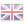Pi and fractal sets
The Mandelbrot set - Dave Boll - Gerald Edgar

A big surprise !

let us consider the point c=(-0.75,X) of the complex plane, that is a point straight over the "neck" of the Mandelbrot set.
Let n be the number of iterations from which the characteristic quadratic sequence of the Mandelbrot set Zn+1=Zn2+c with Z0=-0 diverges (Zn2). With X being smaller and smaller we have:What relation is there between Pi and the Mandelbrot set ?

This pretty picture at the top of the page is the Mandelbrot set (which is ... by the way). It is made up of the points c=x+i*y of the complex plane (with coordinates (x,y) in the catesian plane) such that the sequence Zn+1=Zn2+c with Z0=0 does not diverge.In practice to build up this set and dits graphical representation, one shows that when the modulus of Zn is greater than 2 it will diverge. No need to reach a big value ! The points c of this Mandelbrot set are represented in black (So the points for which Zn stays bounded), and the colours around represent the points according to the value of n from which we consider the sequence diverges.

In 1991 David Bolle tried to verify if the narrowing we can see at (-0.75,0) was actually infinitely thin. That is to say that that however wide a non-zero width vertical line would be passing through that point it would meet the fractal set before the x-axis.

And D Bolle then had the idea of using the point c=(-0.75,X) for the quadratic iteration and to make X tend to 0. And there, what was his surprise when he counted the number of iterations before which the series diverged and by discovering the following table .:

X iterations
1.0 3
0.1 33
0.01 315
0.001 3143
0.0001 31417
0.00001 314160
0.000001 3141593
0.0000001 31415928

Yes, it was Pi that was appearing magnificientely ! As he could not manage to prove this he posted it in 1992 on the sci.maths newsgroup. Gerald Edgar from a university of Ohio answered it on 27 march 1992 by bringing an intuitive explanation of this result. This has been put lower down in the "Trial" section.

After this David Bolle approached the problem with another point c=(0.25+X,0) which were those of the... well... butt part of the set on the right.
Once again, same surprise, this time it is X1/2*n which tends to Pi.

X iterations
1.0 2
0.1 8
0.01 30
0.001 97
0.0001 312
0.00001 991
0.000001 3140
0.0000001 9933
0.00000001 31414
0.000000001 99344
0.0000000001 314157

A few words about Mandelbrot, who started all of it !Benoît Mandelbrot was born in Poland in 1924 and emigrated to France in 1936 with his family, of which Szolem Mandelbrojt, mathematician and professor at the college de France.

Very quickly Benoit is seen as an original mind not really following the trends and ideas of the time. His stay at the ENS Ulm was short (one day!) and his entered Polytechnique in 1944. As opposed to the Bourbakist atmosphere of the French mathematical school he will then spend most of his career in the US (Applied maths and economy in Havard, but also engineering sciences and Physiology at the Yale and Einstein college of medcine !).

His uncle Szolem introduced him in 1945 to the forgotten work of Gaston Julia (1918) about the set of complex points derived drom succesive iterations. Benoit prefered to follow his own path, guided by a wonderful geometrical intuition, but actually gets to meet thework of Julia in the seventies.. He then creates the theory of fractals in "Les objets fractals, forme, hasard et dimension" (1975) and over all "The fractal theory of nature" (1982). Thanks to the research labs that IBM lets him use, he inspires deeply the geometrical vision of fr`ctals and creates the first programs of graphical creation on computers.

A trial to explain the phenomena

It is given by Gerald Elgar and I don't know of any proofs (on internet) of the result. Edgar uses c=(0.25+X,0)

We consider the sequence given by Zn+1=Zn2+1/4+X, with Z0=0.
This sequence increases slowly towards 1/2 (the limit if X=0 following the theorem of the fixed point) then, after this step, diverges faster. We are interested in the point 1/2 by shifting the sequence, i.e. by taking Zn=Yn+1/2. Our equation becomes Yn+1+1/2=(Yn+1/2)2+1/4+X, i.e. :

Y0=-1/2
Yn+1=Yn2+Yn+X

The Yn increase towards 0 slowly around 0, so we can consider that Yn is in this case a function of n, taken as a continuous variable, and - clever part ! - we can consider that Yn+1-Yn is very close to Yn', i.e. Yn differentiated.

So our equation becomes: Yn'=Yn2+X, thus :Hey, we know how to solve this!, it gives :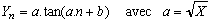But, as we are studying Yn as it goes near 0, the last point of the iteration before Yn escapes corresponds to the moment when tan approaches 0 negatively, i.e. b=0 and a.n close to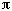i.e :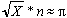where n is the number of iterations before Yn escaped, which is exaclty the experimental result found by D. Bolle.

This metthod is quite surprising as a method to compute Pi. Imagine yourself not calculating iteration after iteration the decimals of Pi but instead its Pi's decimals which will give you the required precision for X ! If we imagined that we could do as many iterations as we wanted in a reasonable time, it could have become a fun game: you can try and found out what the next decimal of Pi is by calculating how many oterations you want thanks to your prevision !

Other cases ?

Lets come back to the first case c=(-0.75,X). We use Zn+1=f(Zn)=Xn2-3/4 woth a fixed point 1/2 with value -1, so we use f(f(z)) and Zn=-1/2+Yn to get Yn close to 0. By the same process as previousely we can find the differential equation :The integral which comes out of this is not easely sovable, but we can derive from it that the required number of iterations for diverence is ... to/(2X). As this concerns the composition f(f(z)), we need this to diverge for f(Zn) to be doubled, i.e/X, which confinrms once again D. Bolle's result for these points.

One of the good principles of this calculation with the Mandelbrot set is that a good number of narrowings exist, we can see it graphically. So several appoaching angles can be used, like the points (-1.25,X). However theree is for this case a chaotic behaviour at the beguining, and my calculator's memory dod not resist ! David Petry, who did the calculation, indicates that n*X/is always very close to an integer or of half an integer for small X. These aapproaching angles are quite obvious because they are vertical or horizontal, but the multiple bulges can give raise to interesting relations.

Even better, we can replace Zn2 by Zn3 or Zn4, this gives new Mandelbrot sets, as the ones below, and here come some new narrowings ! I dont know what points we can try on, if someone can give them to me I can try...(Zn3)(Zn4)

The broad subject of Julia sets

And if you want to go deeper into fractal theory, the yahoo.com directory is quite full. Also there are quite a few Java applets around to draw the Julia and Mandelbrot sets, these are in various sites about fractals.

This theory seems quite fashion nowerdays, especially in france where the TIPE of preparatory schools about dynamic systems during 2 years mention this quite a lot.

To cpmplete the Mandelbrot set and the theory of complex points obtained by iteration let us just briefly talk about the Julia sets which are intimately bounded.

They come from a work by Gaston Julia, brilliant French mathematician between the two wars and professor at the Ecole Polytechnique. We take once again the quadratic iteration but this time its Z0 that we are going to vary. And we take once again the points for which Zn is bounded to Z0 variable to construct the Julia set. THere is tus a Julia set for each point c. ATHis gives new images and new deep properties. For example, if we take a point within the Mandelbrot set, the Mandelbrot set associated is ..., otherwise no. Here are a few examples:Fractal Dendrite, c=(0,1)=i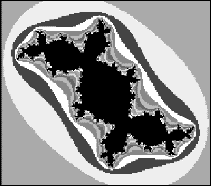DouadysRabbit Fractal c=(-0.123,0.745)=-0.123+0.745iSan Marco Fractalc=(-3/4,0)=-3/4